## Chapter 3 Pair of Linear Equations in Two Variables R.D. Sharma Solutions for Class 10th Math Exercise 3.3

Exercise 3.3

1. Solve the following systems of equations:
11x + 15y + 23 = 0
7x – 2y – 20 = 0

Solution

The given system of equation isHence, the solution of the given system of equations is x = 2,y = - 3.

2. 3x – 7y + 10 = 0
y – 2x – 3 = 0

Solution

The given system of equation isHence, the solution of the given system of equations is  x = -1, y = 1.

3. 0.4x + 0.3y = 1.7
0.7x + 0.2y = 0.8

Solution

The given system of equation isHence, the solution of the given system of equation is x = 2, y = 3.

4. x/2 + y = 0.8

Solution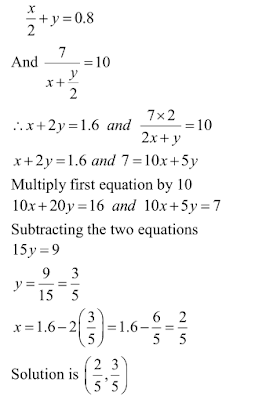5. 7(y + 3) – 2 (x + 3) = 14
4(y – 2) + 3 (x – 3) = 2

Solution

The given system of equations isHence, the solution of the given system of equations is x = 5,y = 1.

6. x/7 + y/3 = 5
x/2 - y/9 = 6

Solution

The given system of equation isHence, the solution of thee given system of equations is  x=14, y=9.

7. x/3 + y/4 = 11
5x/6 - y/3 = 7

Solution

The given system of equations is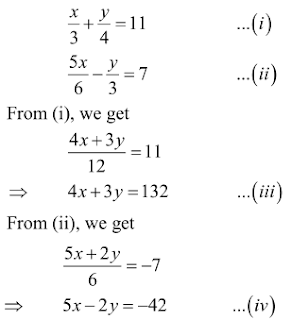Let us eliminate y from the given equations. The coefficients of y in the equations(iii) and
(iv) are 3 and 2 respectively. The L.C.M of 3 and 2 is 6. So, we make the coefficient of y
equal to 6 in the two equations.
Multiplying (iii) by 2 and (iv) by 3, we getHence, the solution of the given system of equations is  x = 6, y = 36.

8. 4u + 3y = 8
6u - 4y = -5

SolutionSo, the solution of the given system of equation is  x=2, y=2.9. x + y/2 = 4
x/3 + 2y = 5

Solution

The given system of equation isHence, solution of the given system of equation is  x=3, y=2.

10. x + 2y = 3/2
2x + y = 3/2

Solution

The given system of equation is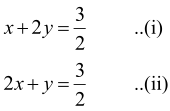Let us eliminate y from the given equations. The Coefficients of y in the given equations
are 2 and 1 respectively. The L.C.M of 2 and 1 is 2. So, we make the coefficient of y equal
to 2 in the two equations.Hence, solution of the given system of equation is x = 1/2, y = 1/2.

11. √2 x + √3 y = 0
√3 x - √8 y = 0

Solution12. 3x - (y+7)/11 + 2 = 10
2y + (x+11)/7 = 10

Solution

The given systems of equation isHence, solution of the given system of equation is  x=3, y=4.

13. 2x - 3/y = 9
3x + 7/y = 2, y ≠ 0

Solution

The given systems of equation is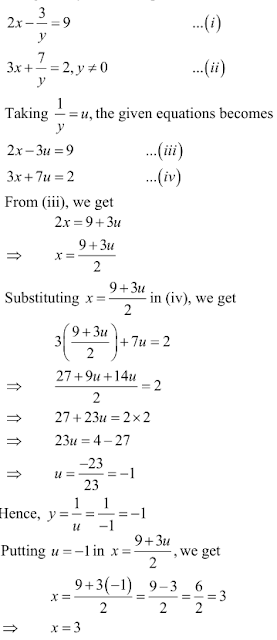Hence, solution of the given system of equation is  x=3,y=1.

14. 0.5x + 0.7y = 0.74
0.3x + 0.5y = 0.5

Solution

The given systems of equations is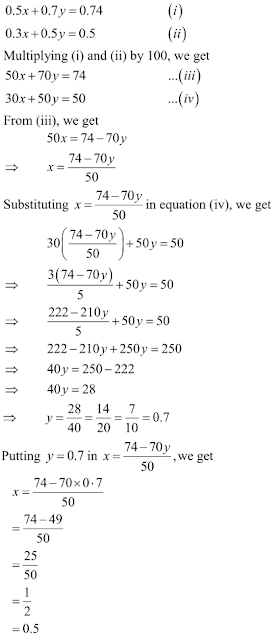Hence, solution of the given system of equation is x = 0 5, y = 0.7.

15 . 1/7x + 1/6y = 3
1/2x - 1/3y = 5

Solution16. 1/2x + 1/3y = 2
1/3x + 1/2y = 13/6

Solution

The given equations are:Multiply equation (i) by 1/2 and (ii) by 1/3 and subtract equation (ii) from (i) we getHence the value of x= 1/2 and y = 1/3 .

17. 15/u + 2v = 17
1/u + 1/v = 36/5

Solution

The given equations are: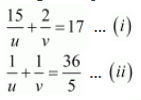Multiply equation (ii) by 2 and subtract (ii) from (i), we getPut the value of u in equation (i), we getHence the value of u=5 and v=1/7

18. 3/x - 1/y = -9
2/x + 3/y = 5

Solution

The given equations are: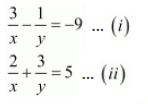Multiply equation (i) by 3 and add both equations, we getPut the value of x in equation(i), we getHence the value of x=1/2 and y=1/3

19. 2/x + 5/y = 1
60/x + 40/y = 19

Solution

The given equations are:Multiply equation (i) by 8 and subtract (ii) from equation (i), we get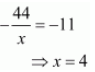Put the value of x in equation(i), we getHence the value x=4 and y=10.

20. 1/5x + 1/6y = 12
1/3x - 3/7y = 8

Solution

The given equations are:Multiply equation (i) by 3/7 and equation (ii) by 1/6, add both equation, we getPut the value of x in equation (i), we get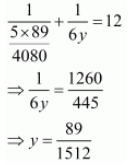Hence the value of = x - 89/4080 and y=89/1512

21. 4/x + 3/y = 14
3/x - 4y = 23

Solution

The given equations are: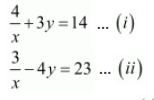Multiply equation (i) by 4 and equation (ii) by 3, add both equations, we get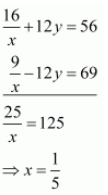Put the value of x in equation (i), we getHence the value of x = 1/5 and y = -2

22. 4/x + 5y = 7
3/x + 4y = 5

Solution

The given equations are: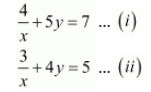Multiply equation (i) by 4 and equation (ii) by 5 and subtract (ii) from (i) we getPut the value of x in equation (i), we get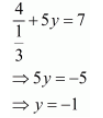Hence the value of x=1/3 and y=-1

23.  2/x + 3/y = 13
5/x - 4y = -2

Solution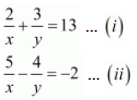Multiply equation (i) by 4 and equation (ii) by 3 and add both equations we getPut the value of x in equation (i), we getHence the value of x=1/2 and y=1/3.

24. 2/√x + 3/√y = 2
4/√x - 9/√y = -1

Solution

The given equations are:Multiply equation (i) by 3 and add both equations we get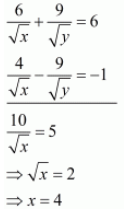Put the value x in equation (i), we getHence the value of x = 4 and y = 9

25. (x+y)/xy = 2
(x-y)/xy = 6

Solution

The given equations are:Put the value y in equation (i), we get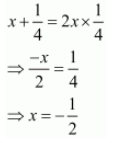Hence the value of x=-1/2 and y=1/4

26. 2/x + 3/y = 9/xy
4/x + 9/y = 21/xy

Solution

The given equations are:Multiply equation (i) by 3 and subtract (ii) from (i), we getPut the value of x in equation (i), we getHence the value of x=1 and y=3 .

27. 6/(x+y) = 7/(x-y) + 3
1/2(x+y) = 1/3(x-y)

Solution

The given equations are: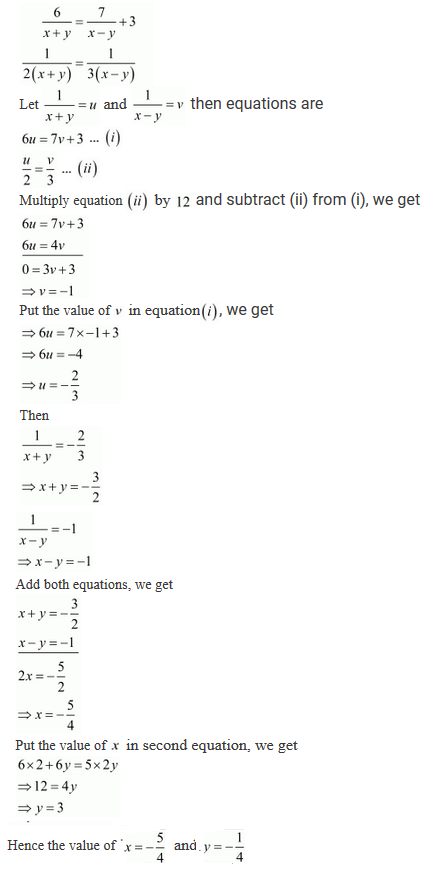28. xy/(x+y) = 6/5
xy/(y-x) = 6

Solution

The given equations are:29. 22/(x+y) + 15/(x-y) = 5
55/(x+y) + 45/(x-y) = 14

Solution

The given equations are:30. 5/(x+y) - 2/(x-y) = -1
15/(x+y) + 7/(x-y) = 10

Solution

The given equations are:31. 3/(x+y) + 2/(x-y) = 2
9/(x+y) - 4/(x-y) = 1

Solution

The given equations are:32. 1/2(x+2y) + 5/3(3x-2y) = -3/2
4(x+2y) - 3/5(3x-2y) = 61/60

Solution

The given equations are:33. 5/(x+1) - 2/(y-1) = 1/2
10/(x+1) + 2/(y-1) = 5/2

Solution

The given equations are:34. x+y=5xy
3x+2y=13xy

Solution

The given equations are:35. x+y = 2xy
x-y/xy = 6

Solution

The given equations are:36. 2(3u-v) = 5uv
2(u+3v) = 5uv

Solution

The given equations are:37. 2/(3x+2y) + 3/(3x-2y) = 17/5
5/(3x+2y) + 1/(3x-2y) = 2

Solution

The given equations are:38. 44/(x+y) + 30/(x-y) = 10
55/(x+y) + 40/(x-y) = 13

Solution

The given equations are:39. 5/(x-1) + 1/(y-2) = 2
6/(x-1) - 3(y-2) = 1
Solution

The given equations are:40. 10/(x+y) + 2/(x-y) = 4
15/(x+y) - 9/(x-y) = -2

Solution

The given equations are:41. 1/(3x+y) + 1/(3x-y) = 3/4
1/2(3x+y) - 1/2(3x-y) = -1/8

Solution

The given equations are:42. (7x-2y)/xy = 5
(8x+7y)/xy = 15

Solution

The given equations are: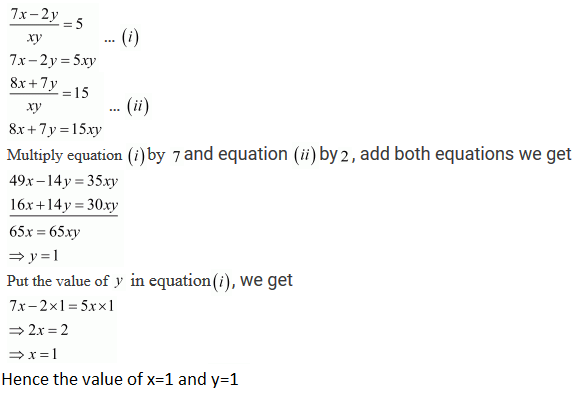43. 152x-378y=-74
-378x + 152y = -604

Solution

The given equations are:44. 99x + 101y = 499
101x + 99y = 501

Solution45. 23x − 29y = 98
29x − 23y = 110

Solution

The given equations are:46. x -y + z = 4
x - 2y - 2z = 9
2x + y + 3z = 1

Solution

The given equations are: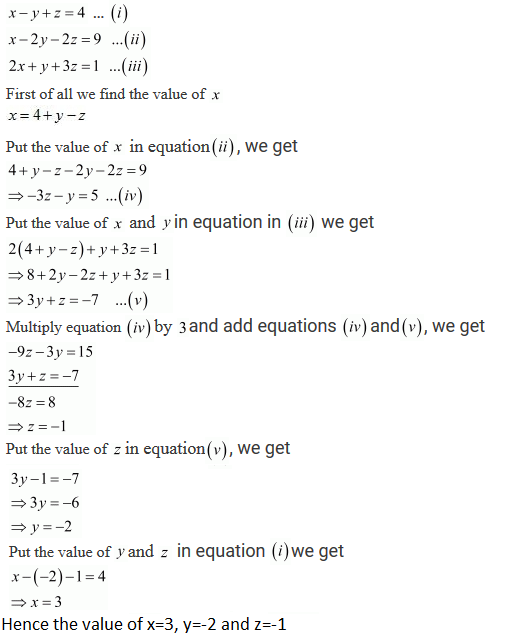47. x − y + z = 4
x + y + z = 2
2x + y − 3z = 0

Solution

The given equations are:48. 21x + 47y = 110
47x + 21y = 162

Solution

21x + 47y = 110 ...(i)
47x + 21y = 162 ...(ii)
Adding (i) and (ii), we get
68x + 68y = 272
⇒ x + y = 4 ...(iii)
Subtracting (i) from (ii), we get
26x - 26y = 52
⇒ x - y = 2 ...(iv)
Adding (iii) and (iv), we get
2x = 6
⇒ x = 3
Putting x = 3 in (iv), we get
3 - y = 2
⇒ y = 1

49. If x+1 is a factor of 2x3 + ax2 + 2bx + 1, then find the values of a and b given that 2a - 3b = 4

Solution

Since (x+1) is a factor of 2x3 + ax2 + 2bx + 1, so
2(-1)3 + a(-1)2 + 2b(-1) + 1 = 0
⇒ -2 + a - 2b + 1 = 0
⇒ a - 2b - 1 = 0
⇒ a - 2b = 1 ...(i)
Also, we are given
2a - 3b = 4 ...(ii)
From (i) and (ii) we get
a = 1 + 2b ...(iii)
Substituting the value of a in (ii), we get
2(1 + 2b) - 3b = 4
⇒ 2 + 4b - 3b = 4
⇒ b = 2
Putting b = 2 in (iii), we get
a = 1 + 2 × 2 = 5
Thus, the value of a = 5 and b = 2.

50. Find the solution of the pair of equations x/10 + y/5 - 1 = 0 and x/8 + y/6 = 15 . Hence, find λ, if y = λ x + 5.

Solution

The given equations areThus, the value of λ = -1/2 .

51. Find the values of x and y in the following rectangle .
Solution

ABCD is the given rectangle. So, AB = CD and AD = BC .
Thus,
x + 3y = 13 ....(i)
3x + y = 7  ....(ii)
Adding (i) and (ii), we get
4x + 4y = 20
⇒ x + y = 5 ....(iii)
Subtracting (i) from (ii), we get
2x - 2y = -6
⇒ x - y = -3 ....(iv)
Adding (iii) and (iv), we get
2x = 2
⇒ x = 1
Putting x = 1 in (iii), we get
1+y = 5
⇒ y = 4
Thus, x = 1 and y = 4.

52. Write an equation of a line passing through the point representing solution of the pair of linear equation x + y = 2 and 2x - y = 1. How many such lines can we find?

Solution

The given equations are
x + y = 2 ....(i)
2x - y = 1 ....(ii)
Adding (i) and (ii), we get
3x = 3
⇒ x = 1
Putting x = 1 in (i), we get
1 + y = 2
⇒ y = 1
Thus, the solution of the given equations is (1, 1).
We know that, infinitely many straight lines pass through a single point.
So, the equation of one such line can be 3x + 2y = 5 or 2x + 3y = 5.

53. Write a pair of linear equations which has the unique solution x = -1,  y = 3. How many such pairs can you write?

Solution

The unique solution is given as x = −1 and y = 3.
The one pair of linear equations having x = −1 and y = 3 as unique solution can be
12x + 5y = 3
2x + y = 1
Similarly, infinitely many pairs of linear equations are possible.